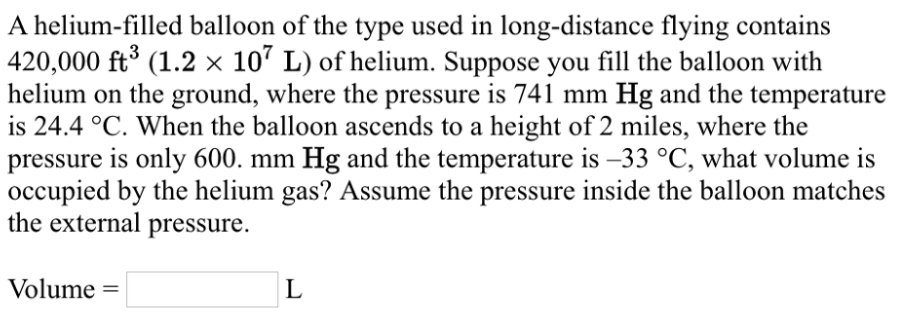# Problem: A helium-filled balloon of the type used in long-distance flying contains 420, 000 ft3 (1.2 times 107 L) of helium. Suppose you fill the balloon with helium on the ground, where the pressure is 741 mm Hg and the temperature is 24.4°C. When the balloon ascends to a height of 2 miles, where the pressure is only 600. mm Hg and the temperature is -33°C, what volume is occupied by the helium gas? Assume the pressure inside the balloon matches the external pressure.

🤓 Based on our data, we think this question is relevant for Professor Staples' class at USM.

###### FREE Expert Solution###### Problem Details

A helium-filled balloon of the type used in long-distance flying contains 420, 000 ft3 (1.2 times 107 L) of helium. Suppose you fill the balloon with helium on the ground, where the pressure is 741 mm Hg and the temperature is 24.4°C. When the balloon ascends to a height of 2 miles, where the pressure is only 600. mm Hg and the temperature is -33°C, what volume is occupied by the helium gas? Assume the pressure inside the balloon matches the external pressure.